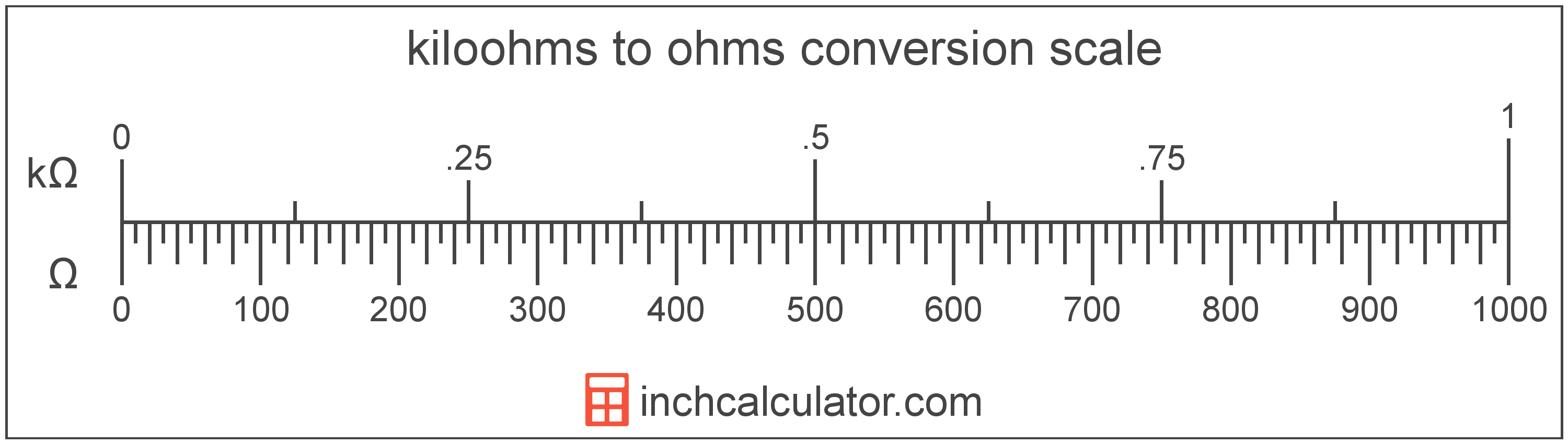# Kiloohms to Ohms Converter

Enter the electrical resistance in kiloohms below to get the value converted to ohms.

Results in Ohms:1 kΩ = 1,000 Ω

Do you want to convert ohms to kiloohms?

## How to Convert Kiloohms to Ohms

To convert a measurement in kiloohms to a measurement in ohms, multiply the electrical resistance by the following conversion ratio: 1,000 ohms/kiloohm.

Since one kiloohm is equal to 1,000 ohms, you can use this simple formula to convert:

ohms = kiloohms × 1,000

The electrical resistance in ohms is equal to the electrical resistance in kiloohms multiplied by 1,000.

For example, here's how to convert 5 kiloohms to ohms using the formula above.
ohms = (5 kΩ × 1,000) = 5,000 Ω### How Many Ohms Are in a Kiloohm?

There are 1,000 ohms in a kiloohm, which is why we use this value in the formula above.

1 kΩ = 1,000 Ω

## What Is a Kiloohm?

One kiloohm is equal to 1,000 ohms. A kiloohm is the resistance across a conductor with an electrical potential difference of 1,000 volts with one ampere of current flowing through it.

The kiloohm is a multiple of the ohm, which is the SI derived unit for electrical resistance. In the metric system, "kilo" is the prefix for thousands, or 103. Kiloohms can be abbreviated as kΩ; for example, 1 kiloohm can be written as 1 kΩ.

## What Is an Ohm?

An ohm is the resistance between two points of an electrical conductor transmitting a current of one ampere when the electrical potential difference is one volt between the points.

The ohm is the SI derived unit for electrical resistance in the metric system. Ohms can be abbreviated as ; for example, 1 ohm can be written as 1 Ω.

### Ohm's Law

Ohm's Law states the current flowing between two points on a conductor is proportional to the voltage and inversely proportional to the resistance. More specifically, the current is equal to the voltage divided by the resistance.

Using Ohm's Law, it's possible to express the resistance in ohms as an expression using current and voltage.

R = VV / IA

Here, the resistance R in ohms is equal to the potential difference V in volts divided by the current I in amperes.

## Kiloohm to Ohm Conversion Table

Table showing various kiloohm measurements converted to ohms.
Kiloohms Ohms
0.001 kΩ 1 Ω
0.002 kΩ 2 Ω
0.003 kΩ 3 Ω
0.004 kΩ 4 Ω
0.005 kΩ 5 Ω
0.006 kΩ 6 Ω
0.007 kΩ 7 Ω
0.008 kΩ 8 Ω
0.009 kΩ 9 Ω
0.01 kΩ 10 Ω
0.02 kΩ 20 Ω
0.03 kΩ 30 Ω
0.04 kΩ 40 Ω
0.05 kΩ 50 Ω
0.06 kΩ 60 Ω
0.07 kΩ 70 Ω
0.08 kΩ 80 Ω
0.09 kΩ 90 Ω
0.1 kΩ 100 Ω
0.2 kΩ 200 Ω
0.3 kΩ 300 Ω
0.4 kΩ 400 Ω
0.5 kΩ 500 Ω
0.6 kΩ 600 Ω
0.7 kΩ 700 Ω
0.8 kΩ 800 Ω
0.9 kΩ 900 Ω
1 kΩ 1,000 Ω

## References

1. International Bureau of Weights and Measures, The International System of Units, 9th Edition, 2019, https://www.bipm.org/documents/20126/41483022/SI-Brochure-9-EN.pdf